## 3.3.2 Angular Dependence

The secondary electron yield is expected to depend on the angle of incidence. Results for the angular dependence for CVD diamond, WMgF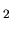TiO, and W(110) samples are presented in Figure 3.6 to 3.9. These samples show no time dependence of the secondary electron yield.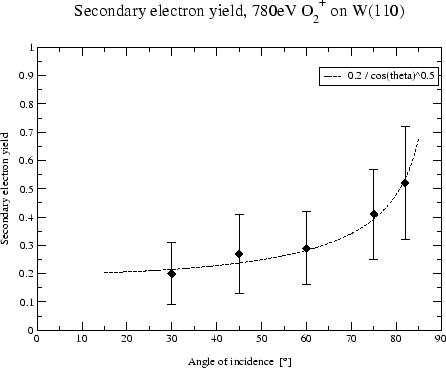Surfaces with a MgO layer exhibited a decrease in the secondary electron yield with time rendering the results for the angular resolved measurements unreliable. Time resolved measurements for these surfaces are presented in the next section.

A model [14,20] assuming a constant energy deposition over the path length within the electron escape zone predicts the secondary electron yield to vary with the impact angle as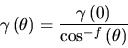(3.13)

where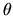is the angle of incidence measured from the surface normal and the exponent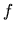an empirical constant around 1. This model is derived from proton bombardment at energies above a few keV. In my measurements the secondary electron yield was normally higher at higher angles of incidence except for samples showing a time dependence where no useful results with angular resolution were obtained. Figure 3.9 depicts an angular resolved measurement of a tungsten surface with more data points than usually taken. A fit using Equation 3.13 yields a value forof 0.5. The same value was found by . But this value is probably only of limited use because the secondary electron yields near an angle of incidence of 90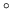are probably overestimated due to the ionization efficiency. The ionization efficiency can no longer be neglected at these angles as an ionization efficiency of up to 20% is expected and the secondary electron yield is of the same order.

To summarize, no simple dependence on the angle of incidence as suggested by Equation 3.13 valid for all surfaces and all energies of interest in this project could be observed but generally the yield increased with energy and towards higher incidence angles.

March 2001 - Martin Wieser, Physikalisches Institut, University of Berne, Switzerland